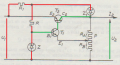# voltage Stability factor of voltage regulation circuits

#### Jamiel5600

Joined Jan 19, 2021
3

#### AlbertHall

Joined Jun 4, 2014
12,199
Without Z1, the current through zener Z would change as Ui changes and so produce some change in the reference voltage.
Z1 keeps the voltage at the junction of R1 and R constant(ish) and the voltage across R will change less when Ui changes and so the voltage across Z will be more constant.

•DickCappels

#### Jamiel5600

Joined Jan 19, 2021
3
Without Z1, the current through zener Z would change as Ui changes and so produce some change in the reference voltage.
Z1 keeps the voltage at the junction of R1 and R constant(ish) and the voltage across R will change less when Ui changes and so the voltage across Z will be more constant.
Thank You Albert Hall

if i've well understood , i will say that Z1 must drain overcurrent caused by the increasing of U1 to maintain the voltage at the node of the junction R-R1 constant ,

How to turn this into equations between U1 and U2.

#### AlbertHall

Joined Jun 4, 2014
12,199
Thank You Albert Hall

if i've well understood , i will say that Z1 must drain overcurrent caused by the increasing of U1 to maintain the voltage at the node of the junction R-R1 constant ,

How to turn this into equations between U1 and U2.

#### Jamiel5600

Joined Jan 19, 2021
3
Thank You Albert Hall

if i've well understood , i will say that Z1 must drain overcurrent caused by the increasing of U1 to maintain the voltage at the node of the junction R-R1 constant ,

How to turn this into equations between U1 and U2.
For Example for the feedback through Ra-Rb potentiometer ,

we have Vb of T1 => Vb1=( Vz - Ib1 r1) and Ve of T1 => Ve1=( V2( Rb/Ra+Rb))

T1 conduct if Vbe (T1) >= 0,7 so we have Vbe(T1) = (Vz-Ib1*r1) - ( U2*(Rb/rb+Ra)) = 0,7 (0*)

let consider the figure belowif you follow the green line we have

U2 = Vz + Ib1 *r1 + Ic1* r2 (1*)

if you follow the red line and uses the MILLMAN Formula ( V = ΣViGi / ΣGi )
we have
Vz = (Rz1*Rz *U1 + R1* Rz*U2) / ( R1*Rz1) + (R1*R) + (R1*Rz) + (Rz1*Rz) +(Rz1*R) (2*)

if you replace the expression of Vz given by the second equation (2*) into the first equation (1*) , i have my relation between U1 and U2

But i've to calculate Ib1 , and for this i'm feel lost

But i think that we could use our first equation (0*) to eliminate Ib1 in our system Equations

given by

Vbe(T1) = (Vz-Ib1*r1) - ( U2*(Rb/rb+Ra)) = 0,7 (0*)